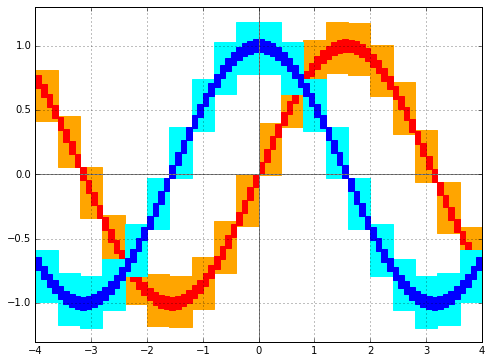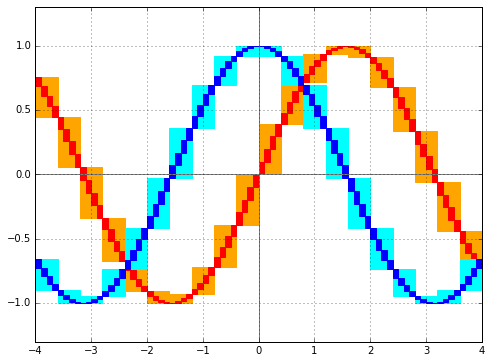# Arb 2.14 released

July 22, 2018

I have just issued version 2.14 of Arb. As usual, downloads are available via GitHub, and there is an extensive changelog in the documentation. This post presents some highlights.

## Improved linear algebra

Matrix multiplication, linear solving, inverse and determinant computation have been improved significantly, enabling factor 10-100 speedups with large matrices. This is all described in two previous blog posts:

## Tighter enclosures

Continuing a project started in Arb 2.13, more functions (including trigonometric functions, hyperbolic functions, and complex reciprocal square roots) have been optimized to produce tighter enclosures when given wide input intervals. Many of these functions have also been optimized to adjust the precision automatically based on the input accuracy: if the function gets called with 1000-bit precision but the output only can be accurate to 100 bits due to the radius of the input, only about 100 bits will be used internally.

The following plots show the enclosures for sine and cosine on intervals of width 0.1 and 0.4, with Arb 2.13 on the left and Arb 2.14 on the right:Optimizing the enclosures for wide intervals speeds up many algorithms that depend on bounding the range of a function, such as root isolation and integration. For example, running the real_roots program to isolate the zeros of the Riemann zeta function on $0.5 + [0,100]i$, we get the following results:

Arb 2.13 Arb 2.14
> build/examples/real_roots 0 0 100 -verbose
interval: [0, 100]
maxdepth = 30, maxeval = 100000, maxfound = 100000, low_prec = 30
found isolated root in: [14.111328125, 14.16015625]
found isolated root in: [20.99609375, 21.044921875]
found isolated root in: [25, 25.048828125]
found isolated root in: [30.419921875, 30.4443359375]
found isolated root in: [32.91015625, 32.958984375]
found isolated root in: [37.548828125, 37.59765625]
found isolated root in: [40.91796875, 40.966796875]
found isolated root in: [43.310546875, 43.3349609375]
found isolated root in: [47.998046875, 48.0224609375]
found isolated root in: [49.755859375, 49.7802734375]
found isolated root in: [52.9296875, 52.978515625]
found isolated root in: [56.4453125, 56.494140625]
found isolated root in: [59.326171875, 59.3505859375]
found isolated root in: [60.8154296875, 60.83984375]
found isolated root in: [65.1123046875, 65.13671875]
found isolated root in: [67.0654296875, 67.08984375]
found isolated root in: [69.53125, 69.5556640625]
found isolated root in: [72.021484375, 72.0703125]
found isolated root in: [75.68359375, 75.7080078125]
found isolated root in: [77.1240234375, 77.1484375]
found isolated root in: [79.3212890625, 79.345703125]
found isolated root in: [82.91015625, 82.9345703125]
found isolated root in: [84.716796875, 84.7412109375]
found isolated root in: [87.40234375, 87.4267578125]
found isolated root in: [88.7939453125, 88.818359375]
found isolated root in: [92.48046875, 92.5048828125]
found isolated root in: [94.62890625, 94.6533203125]
found isolated root in: [95.849609375, 95.8740234375]
found isolated root in: [98.828125, 98.876953125]
---------------------------------------------------------------
Found roots: 29
Subintervals possibly containing undetected roots: 0
Function evaluations: 7938
cpu/wall(s): 0.613 0.614
virt/peak/res/peak(MB): 26.66 26.66 5.17 5.17

> build/examples/real_roots 0 0 100 -verbose
interval: [0, 100]
maxdepth = 30, maxeval = 100000, maxfound = 100000, low_prec = 30
found isolated root in: [14.0625, 14.16015625]
found isolated root in: [20.99609375, 21.09375]
found isolated root in: [25, 25.048828125]
found isolated root in: [30.419921875, 30.46875]
found isolated root in: [32.91015625, 32.958984375]
found isolated root in: [37.548828125, 37.59765625]
found isolated root in: [40.91796875, 40.966796875]
found isolated root in: [43.310546875, 43.359375]
found isolated root in: [47.998046875, 48.046875]
found isolated root in: [49.755859375, 49.8046875]
found isolated root in: [52.9296875, 52.978515625]
found isolated root in: [56.4453125, 56.54296875]
found isolated root in: [59.326171875, 59.3505859375]
found isolated root in: [60.791015625, 60.83984375]
found isolated root in: [65.087890625, 65.13671875]
found isolated root in: [67.041015625, 67.08984375]
found isolated root in: [69.53125, 69.580078125]
found isolated root in: [72.021484375, 72.0703125]
found isolated root in: [75.68359375, 75.732421875]
found isolated root in: [77.099609375, 77.1484375]
found isolated root in: [79.296875, 79.345703125]
found isolated root in: [82.91015625, 82.958984375]
found isolated root in: [84.716796875, 84.765625]
found isolated root in: [87.40234375, 87.4267578125]
found isolated root in: [88.76953125, 88.818359375]
found isolated root in: [92.48046875, 92.529296875]
found isolated root in: [94.62890625, 94.6533203125]
found isolated root in: [95.849609375, 95.8740234375]
found isolated root in: [98.828125, 98.876953125]
---------------------------------------------------------------
Found roots: 29
Subintervals possibly containing undetected roots: 0
Function evaluations: 4773
cpu/wall(s): 0.372 0.373
virt/peak/res/peak(MB): 26.86 26.86 5.24 5.24


The new version requires 1.6 times fewer function evaluations (and runs 1.6 times faster).

Another example is evaluating $$\int_0^{\pi} \frac{x \sin(x)}{1+\cos^2(x)} dx$$ with integrals.c, at the default 64-bit precision:

Arb 2.13 Arb 2.14
fredrik@agm:~/src/arbold/arb-2.13.0$build/examples/integrals -i 31 -verbose I31 = int_0^pi x sin(x) / (1 + cos(x)^2) dx ... depth 5/128, eval 373/68096, 14 leaf intervals cpu/wall(s): 0.004 0.004 I31 = [2.4674011002723397 +/- 5.88e-17]  fredrik@agm:~/src/arb$ build/examples/integrals -i 31 -verbose
I31 = int_0^pi x sin(x) / (1 + cos(x)^2) dx ...
depth 3/128, eval 159/68096, 6 leaf intervals
cpu/wall(s): 0.003 0.002
I31 = [2.4674011002723397 +/- 5.43e-17]


Here Arb 2.14 requires a whopping 2.3 times fewer function evaluations.

## Stieltjes constants

This is a relatively esoteric feature, but I will mention it here since it is the result of a small research project, documented in a joint paper with Iaroslav Blagouchine (University of Toulon):

The (generalized) Stieltjes constants $\gamma_n(a)$ are essentially the Laurent series coefficients of the Hurwitz zeta function about its pole at $s = 1$, that is, $$\zeta(s,a)=\frac{1}{s-1}+\sum_{n=0}^\infty \frac{(-1)^n}{n!} \gamma_n(a) (s-1)^n.$$ It was previously possible to compute these coefficients with Arb by simply evaluating the Hurwitz zeta function at the power series $s = 1+x+O(x^{n+1})$, but this gets very slow for large orders $n$. The new method acb_dirichlet_stieltjes computes an isolated Stieltjes constant $\gamma_n(a)$ in time that only grows logarithmically with $n$. It achieves this by using the rigorous numerical integration code in Arb to evaluate an integral representation along a well-chosen complex contour as described in the paper.

As an example, here is $\gamma_{10^{4}}(1)$ evaluated at 333-bit precision, which takes 0.025 seconds:

[-2.210497056722106086297108285753650190023439717472940051038769914291165296866618985281889361328929699e+6883 +/- 2.71e+6783]

Here is the gigantic $\gamma_{10^{100}}(1)$ evaluated at 333-bit precision, which takes 1.5 seconds:

[3.187431418702399279997416469927116651394309910883846922507106265983048934155937559668288022632306095e+23463942922772540809493678383990911609034476898698373852057791115792156640521582344171254175433483694 +/- 3.97e+23463942922772540809493678383990911609034476898698373852057791115792156640521582344171254175433483594]

Here is $\gamma_{10^{30}}(2+3i)$ evaluated at 333-bit precision, which takes 0.4 seconds:

gamma_10^30(2+3i) = [-5.46826056593866267752220079049822468225110689141600287580368903467405792782955102176627531733819922e+1793244444699276018580262442765 +/- 2.84e+1793244444699276018580262442666] + [4.56767759461445914483181980735214745595380177200701058136229790477802429634348195941046788610035824e+1793244444699276018580262442765 +/- 5.23e+1793244444699276018580262442666]*I

Unfortunately, I noticed when writing this that the fast algorithm is not used when $a \ne 1$ in Arb 2.14.0 (although it can be called manually). Argh! This will be fixed in an imminent 2.14.1 patch release, along other issues users may point out in the next couple of days.